# Style

View as Grid List

Items 1-36 of 227

Page
per page
Set Descending Direction
1.As low as \$295.00
2.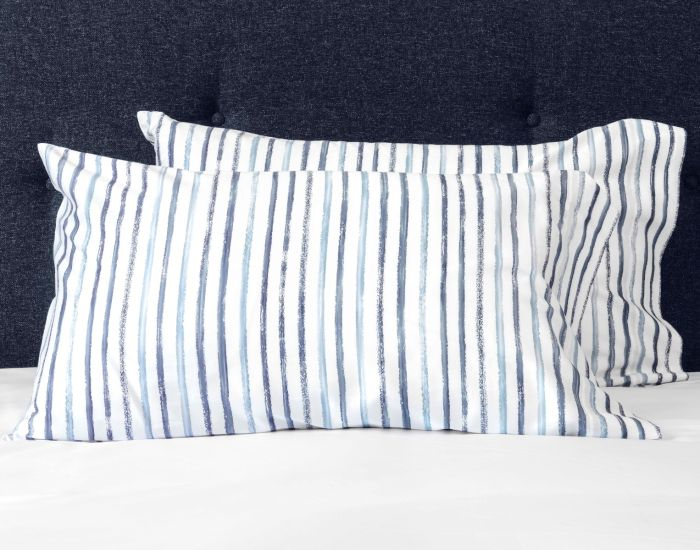As low as \$155.00
3.As low as \$299.00
4.As low as \$75.00
5.As low as \$465.00
6.As low as \$295.00
7.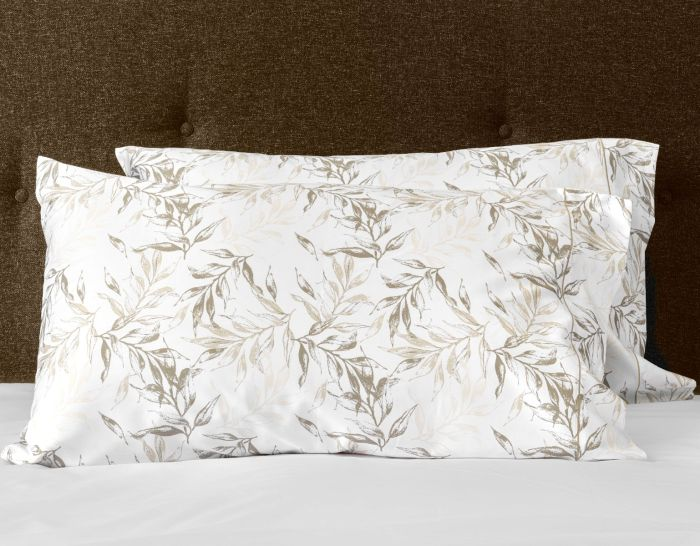As low as \$175.00
8.As low as \$295.00
9.As low as \$95.00
10.As low as \$298.00
11.As low as \$695.00
12.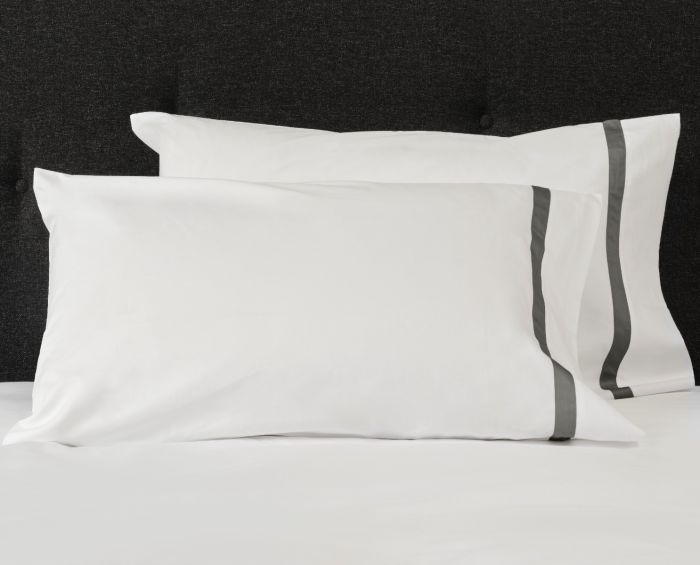As low as \$165.00
13.As low as \$95.00
14.As low as \$595.00
15.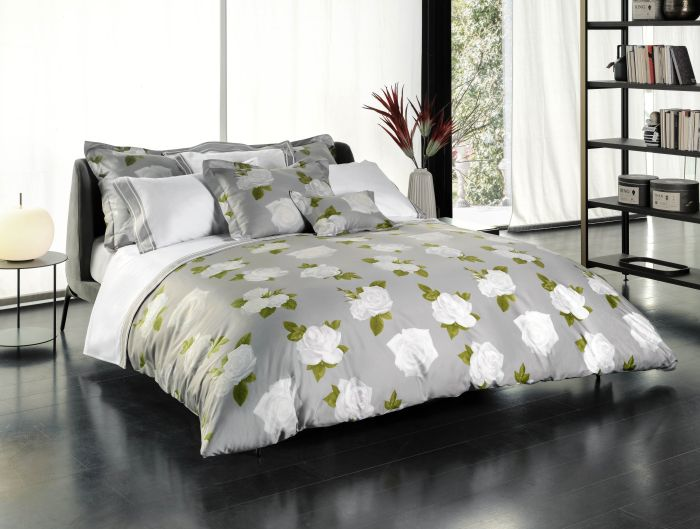As low as \$465.00
16.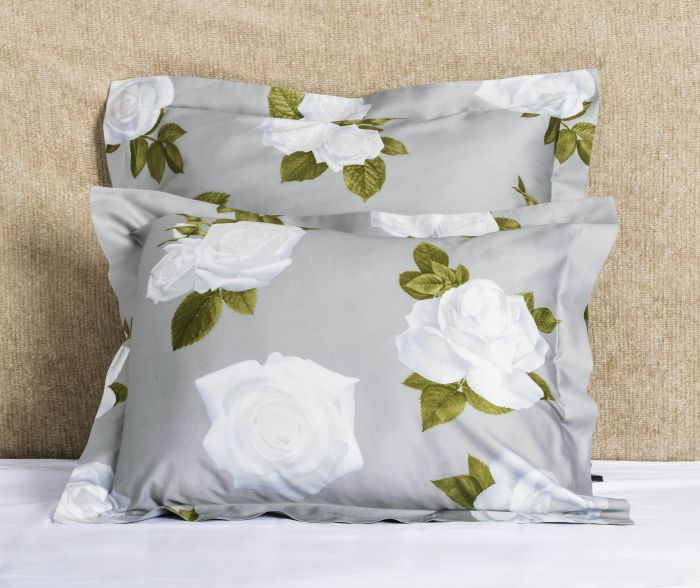As low as \$140.00
17.As low as \$465.00
18.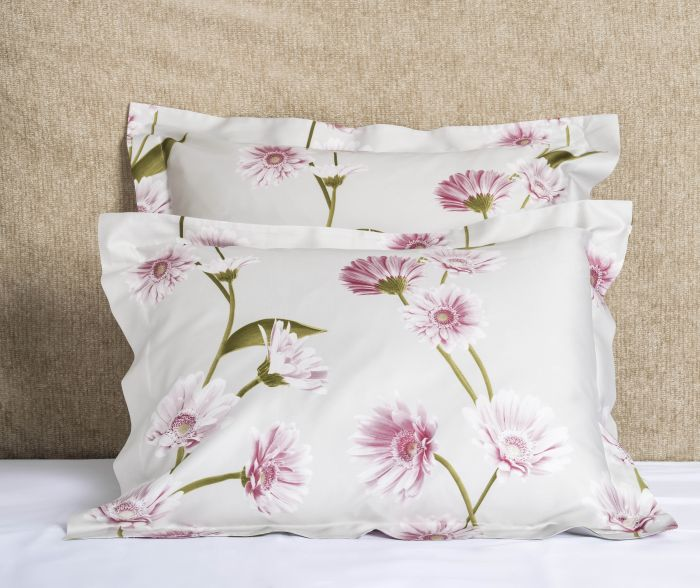As low as \$140.00
19.As low as \$690.00
20.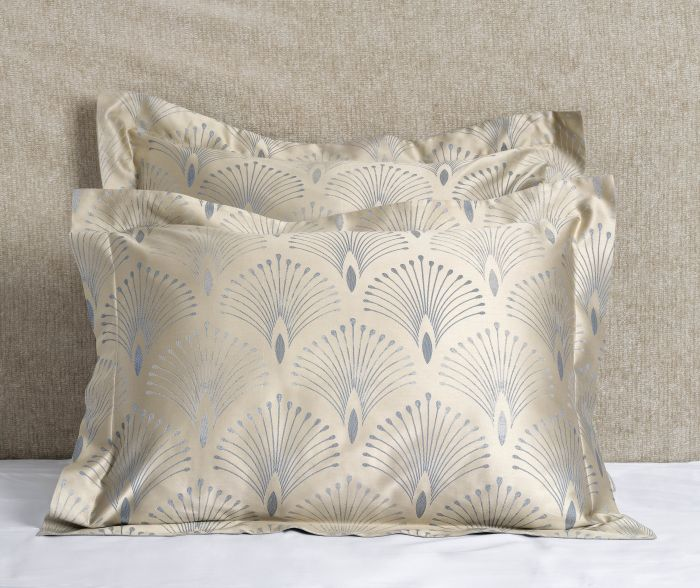As low as \$180.00
21.As low as \$575.00
22.As low as \$900.00
23.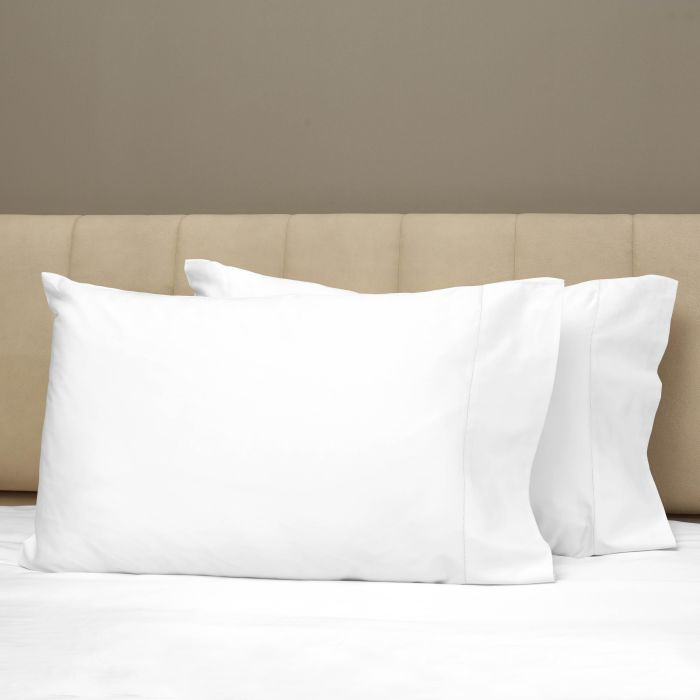As low as \$210.00
24.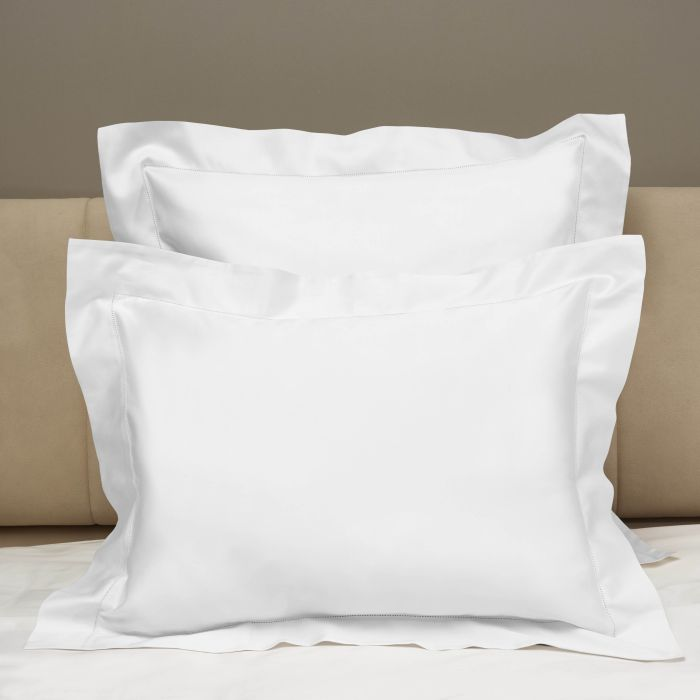As low as \$180.00
25.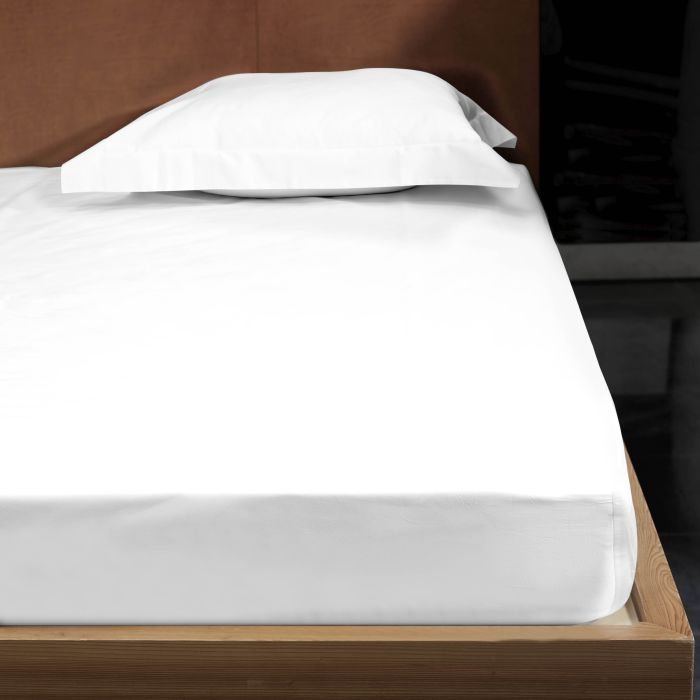As low as \$285.00
26.As low as \$320.00
27.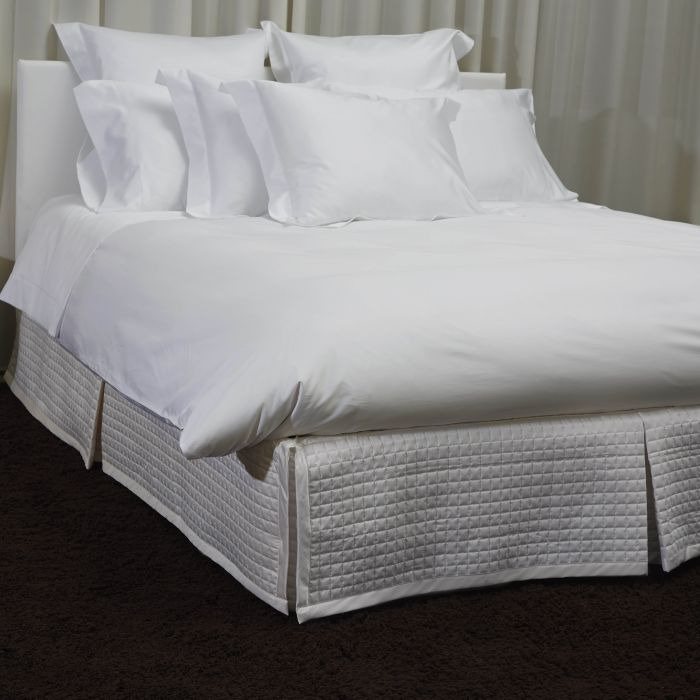As low as \$730.00
28.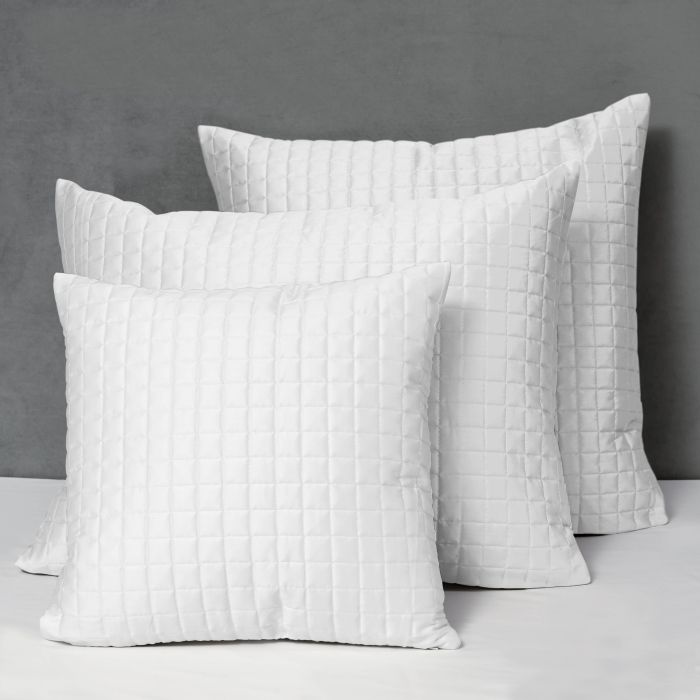As low as \$85.00
29.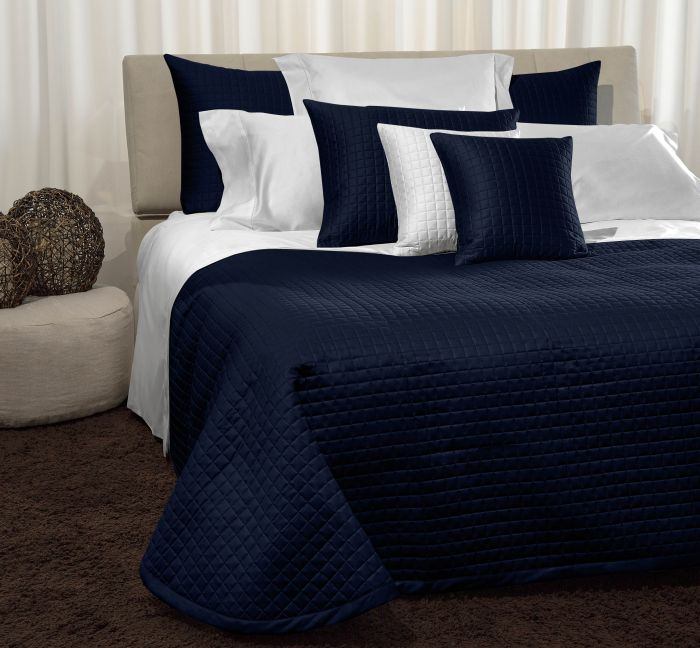As low as \$425.00
30.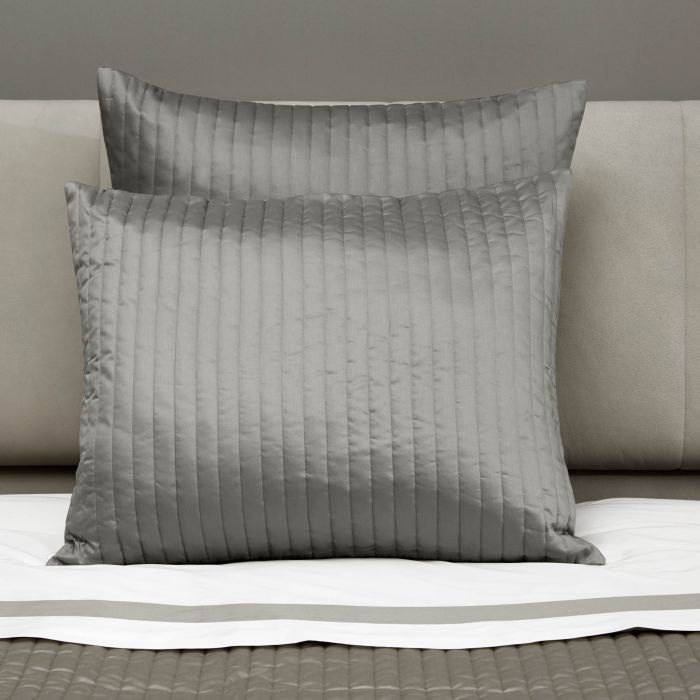As low as \$95.00
31.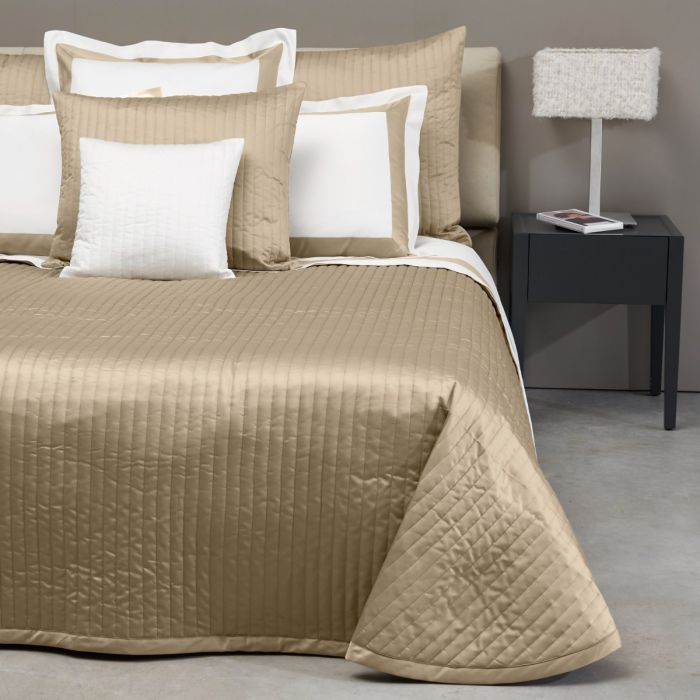As low as \$515.00
32.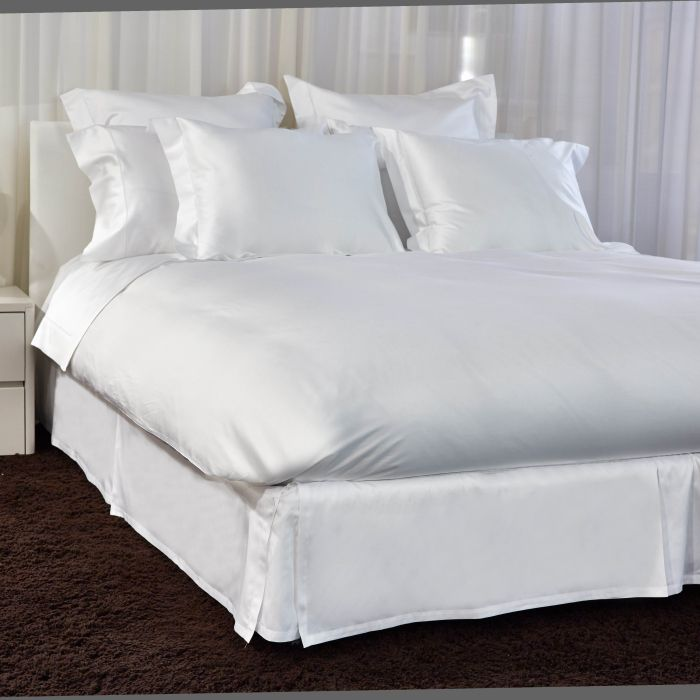As low as \$400.00
33.As low as \$400.00
34.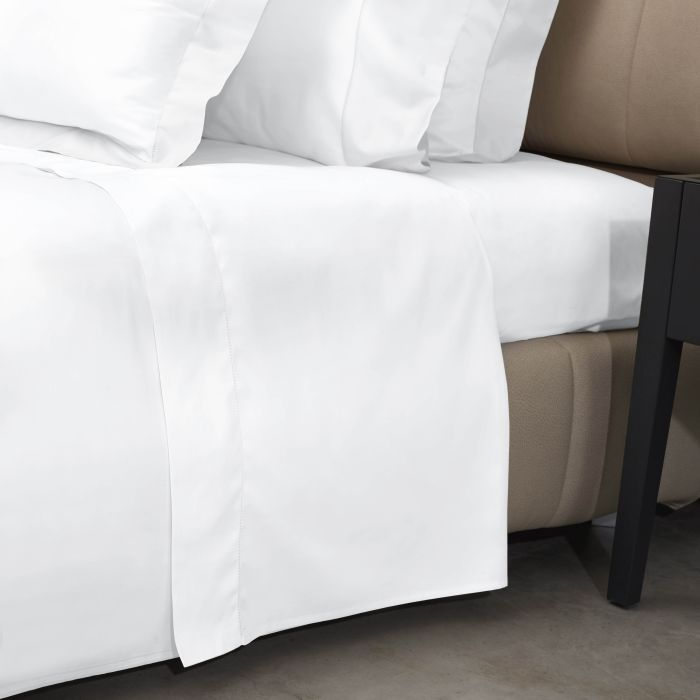As low as \$435.00
35.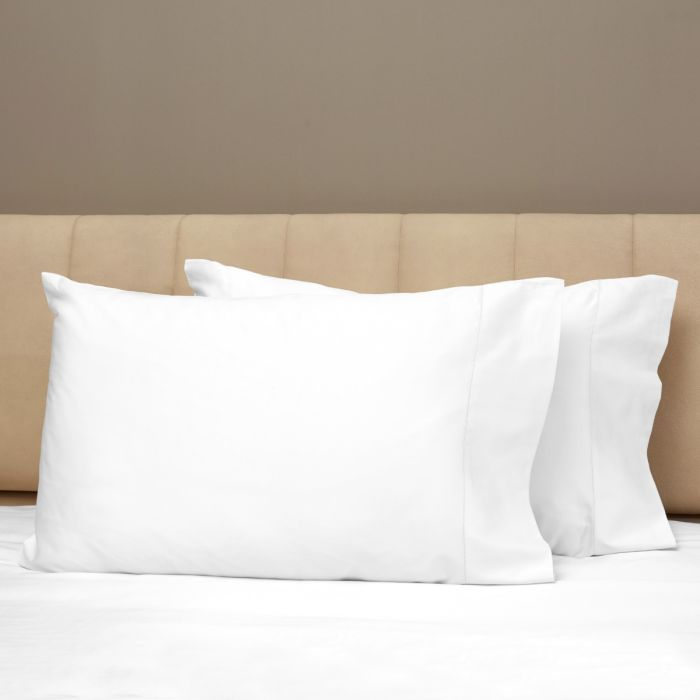As low as \$130.00
36.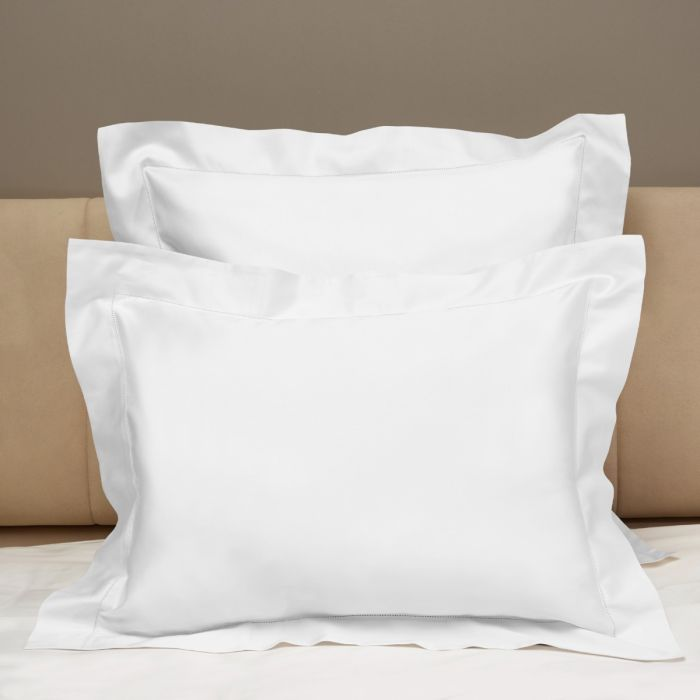As low as \$135.00
View as Grid List

Items 1-36 of 227

Page
per page
Set Descending Direction

Any reference to thread count (TC) refers to the commonly accepted commercial definition of the number of yarns per square inch including 2 ply yarns for obtaining total thread count. It is not a reference to the “technical” definition as promulgated by ASTM Standard D 3775 which considers 2 or more ply yarns as one thread or end. Applying D 3775 to multiple ply yarns will result in a lower technical thread count than the commercial definition.